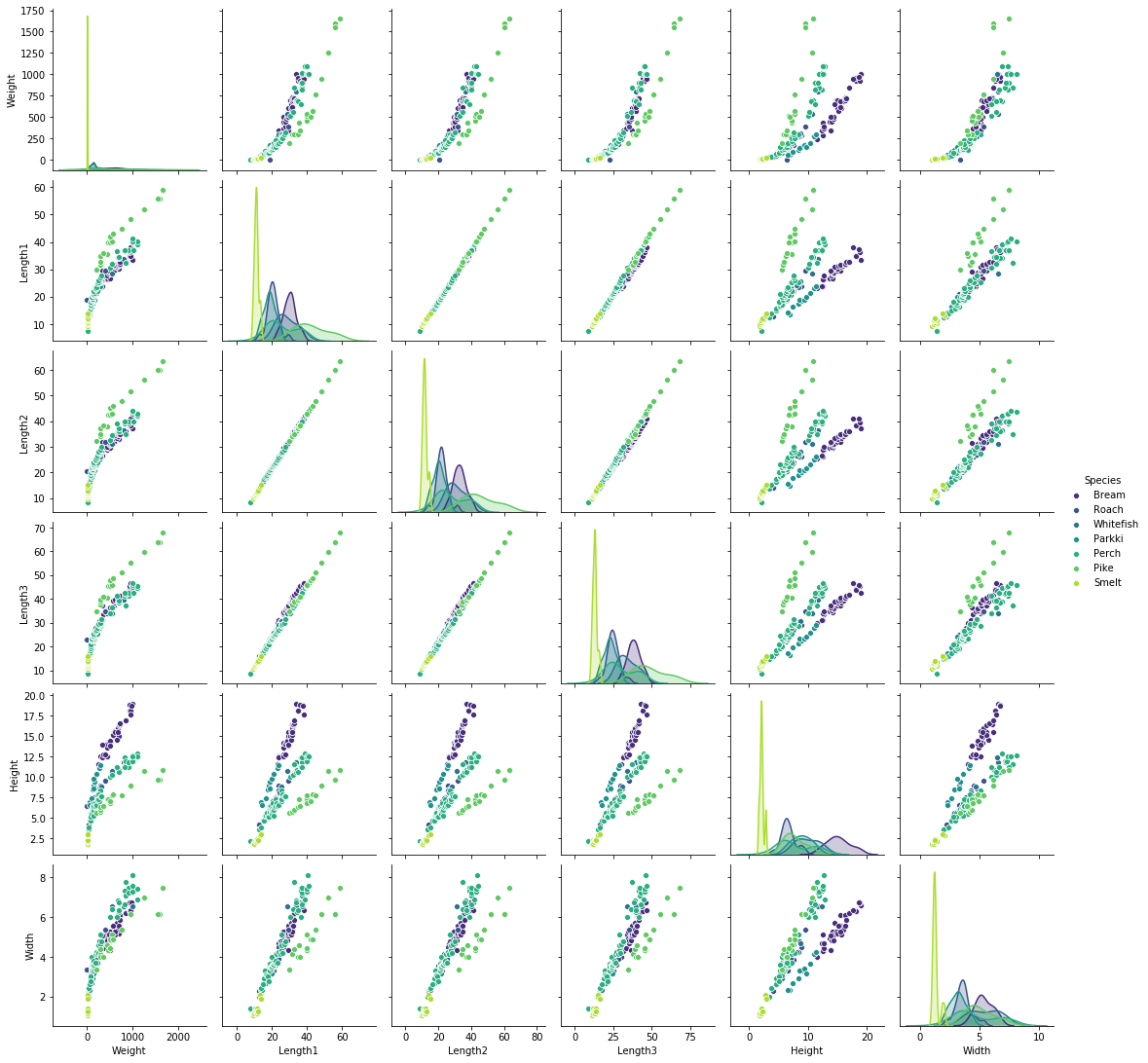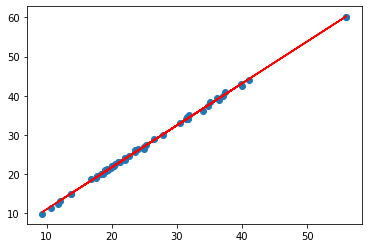# Machine Learning 101: Linear Regression in PythonLinear Regression is probably the first ML algorithm any Data Scientist will encounter in its journey. As a matter of fact it is the easiest ML algorithm to learn conceptually. Let’s take a look.

## Classification vs Regression

Machine Learning essentially deals with two kinds of problems:

• Classification: predicting a class, for example whether a user is male or female (the two classes) given their history of purchased items.
• Regression: predicting a value, for example the price (the value) of a used car given the model, the age, the kilometers on the odometer.

It is important to remember that Machine Learning is no magic, ML algorithms are still algorithms: multiple inputs, one output. The most important difference between a traditional algorithm and an ML one is the “experience” the ML algorithm gains during the training phase.

In Classification problems the algorithm tries to predict the class the entry will fall into, it may be two classes (such as the example above, male versus female) or more than two classes. The former is often called Binary Classification the latter is referred to as Multiclass Classification.

In Regression there is no class to predict, instead there is a scale and the algorithm tries to predict the value on that scale. In the example above the price is the sought value.

## Linear Regression in Python

Linear Regression is the most basic algorithm of Machine Learning and it is usually the first one taught. Linear Regression is usually applied to Regression Problems, you may also apply it to a classification problem, but you will soon discover it is not a good idea. Although the term may seem fancy, the idea behind it is pretty easy to understand.

Let’s suppose we have two variables X and y, imagine that X and y grow at the same rate: adding 1 to X means that also y grows by 1. If you draw a plot of these two variables you will have a straight line. Knowing the equation of that line (y = mX + q) will enable you to know y if you know X.

Linear Regression is about finding that line from a number of observations (X and y). As with every ML algorithm, the more observations you have, and the more accurate they are, the better the algorithm will be at predicting the outcome.

## Linear Regression using fish (regression problem)

The following notebook uses the Fish market dataset available here, it is free and released under the GPL2 license. This dataset includes a number of species of fish and for each fish some measurements such as weight and height.

### (Basic) Explanatory Data Analysis

Every good ML algorithm should start with an in-depth Explanatory Data Analysis (EDA). In the EDA you should always try to explore the data as much as possible, through exploration it is possible to infer basic features of the data, from those basic inferences you can start developing a basic intuition. From there you can start formulating hypotheses and implement the algorithm you see fit.

As the purpose of this notebook is to illustrate the Linear Regression applied to a Regression Problem, the performed EDA will outline just the basic features of the dataset.

Firstly, let's import basic utilities:

In :
%matplotlib inline
import pandas as pd
import numpy as np
import matplotlib.pyplot as plt
import seaborn as sns

np.random.seed(101) # This is needed so that if you run this notebook again you will get the same results


Let's now read the csv file (keep in mind it should be in the same folder as the notebook!):

In :
df = pd.read_csv('Fish.csv')


Let's take a look at the first rows of the dataset. It is important to do this in order to get a basic understanding.

In :
df.head()

Out:
Species Weight Length1 Length2 Length3 Height Width
0 Bream 242.0 23.2 25.4 30.0 11.5200 4.0200
1 Bream 290.0 24.0 26.3 31.2 12.4800 4.3056
2 Bream 340.0 23.9 26.5 31.1 12.3778 4.6961
3 Bream 363.0 26.3 29.0 33.5 12.7300 4.4555
4 Bream 430.0 26.5 29.0 34.0 12.4440 5.1340

Now let's take a closer look to the dataset to get important statistical indicators such as the mean and standard deviation

In :
df.describe()

Out:
Weight Length1 Length2 Length3 Height Width
count 159.000000 159.000000 159.000000 159.000000 159.000000 159.000000
mean 398.326415 26.247170 28.415723 31.227044 8.970994 4.417486
std 357.978317 9.996441 10.716328 11.610246 4.286208 1.685804
min 0.000000 7.500000 8.400000 8.800000 1.728400 1.047600
25% 120.000000 19.050000 21.000000 23.150000 5.944800 3.385650
50% 273.000000 25.200000 27.300000 29.400000 7.786000 4.248500
75% 650.000000 32.700000 35.500000 39.650000 12.365900 5.584500
max 1650.000000 59.000000 63.400000 68.000000 18.957000 8.142000

Let's now plot each numerical feature against each other, in order to get a clear distinction use a palette with high contrast (Viridis) and use the species as color.

In :
sns.pairplot(df, hue='Species', palette='viridis')

Out:
<seaborn.axisgrid.PairGrid at 0x1f7b30cd188>As you can see there is quite a strong pattern between: Length1 and Length2, Length2 and Length3, Length3 and Length1. This is prefect to get started. What kind of secrets does these three lengths hide? Are you able to speculate about what Length1 and Lenght2 are?

### Linear Regression (one independent variable): Let's predict Length2 Knowing Length1

It is important to reshape the two dimensions (X and y), if you don't do this the model will throw an error.

In :
X = np.array(df['Length1']).reshape(-1, 1)
y = np.array(df['Length2']).reshape(-1, 1)


Split the dataset in two parts: train and test. This is needed to calculate the accuracy (and many other metrics) of the model. We will use the train part during the training, and the test part during the evaluation. The model will not see the test part during its training.

In :
from sklearn.model_selection import train_test_split
X_train, X_test, y_train, y_test = train_test_split(X, y, test_size = 0.33, random_state=101)


Import the model and instantiate it:

In :
from sklearn.linear_model import LinearRegression

lr = LinearRegression()


Now let's train the model:

In :
lr.fit(X_train, y_train)

Out:
LinearRegression(copy_X=True, fit_intercept=True, n_jobs=None, normalize=False)

Let's review how the model is doing using the R2. R2 is a statistical indicator to know whether the model is "a good fit" and how well it performs. In this case (one independent variable) the R2 is equal to the Pearson Correlation Coefficient. R2 can assume values between 0.0 and 1.0, where 0 means the worst fit and 1 the best fit.

In :
lr.score(X_test, y_test)

Out:
0.9990508254380637

Pretty high! That's due to the fact the two variables (Length1 and Length2) take the shape of a straight line as observed during the EDA. Let's now plot the predict values against the test part of the dataset.

In :
plt.scatter(X_test, y_test)
plt.plot(X_test, lr.predict(X_test), color='red')
plt.show()As you can see the line is pretty close, athough not perfect. But how come these two?

#### Conclusion: Let's take a step back

"Are you able to speculate about what Length1 and Lenght2 are?"

You followed the notebook up to now without knowing what Length1 and Lenght2 are. Let's take a step back.

Length1 represents Vertical length in centimeters, while Lenght2 is Diagonal length in centimeters, kudos if you get that right without reading the solution.

If you look at it now it is just natural to think "If the fish is inscribed within a rectangle, the more the diagonal grows the more the height of the rectangle will grow.

### Linear Regression (multiple independent variables): Let's predict weight

The procedure to predict the weight of the fish using Linear Regression is pretty similar to last one. The only notable difference is that there are multiple independent variables. Since it is not the purpose of this notebook to explain how to represent categorical variables to use them with a ML model, the "Species" variable will be dropped entirely (will not contribute).

In :
X = df.drop(['Weight', 'Species'], axis=1)
y = df['Weight']

In :
from sklearn.model_selection import train_test_split
X_train, X_test, y_train, y_test = train_test_split(X, y, test_size = 0.33, random_state=101)

In :
from sklearn.linear_model import LinearRegression

lr = LinearRegression()

In :
lr.fit(X_train, y_train)

Out:
LinearRegression(copy_X=True, fit_intercept=True, n_jobs=None, normalize=False)
In :
lr.score(X_test, y_test)

Out:
0.8642224833122479

As you can see the R2 score is not as high as it was earlier, yet it is still pretty high. Since it is pretty difficult to show the predicted values against the actual values, let's take a look at them as they are.

In :
df_t = df.copy()

In :
df_t['Predicted Weight'] = lr.predict(df_t.drop(['Weight', 'Species'], axis=1))

In :
df_t['Difference'] = df_t['Weight'] - df_t['Predicted Weight']

In :
df_t[['Weight', 'Predicted Weight', 'Difference']].head(20)

Out:
Weight Predicted Weight Difference
0 242.0 329.661992 -87.661992
1 290.0 374.756426 -84.756426
2 340.0 384.719326 -44.719326
3 363.0 427.226647 -64.226647
4 430.0 463.235780 -33.235780
5 450.0 465.648356 -15.648356
6 500.0 503.924833 -3.924833
7 390.0 470.949250 -80.949250
8 450.0 509.362339 -59.362339
9 500.0 541.636382 -41.636382
10 475.0 535.761445 -60.761445
11 500.0 542.386113 -42.386113
12 500.0 511.929040 -11.929040
13 340.0 551.704054 -211.704054
14 600.0 577.196006 22.803994
15 600.0 612.411903 -12.411903
16 700.0 600.716507 99.283493
17 700.0 593.223367 106.776633
18 610.0 625.015601 -15.015601
19 650.0 636.927079 13.072921

### Conclusion: The model is a good fit, but is it the best it can do?

The model is not performing well (or rather, not as well as hoped) for this problem and data. The predicted weight (in grams) is sometimes really close (-4) and sometimes far off (-212).

The EDA didn't cover some basics such as feature selection and removing outliers. Also the model was deprived of a feature: the Species which, as you might imagine, may influence the weight of a fish. Another important factor is the size of the dataset: usually larger datasets lead to more accurate results given that data is not trash.

Even though R2 is ~0.85, the model is not a good fit at predicting this value. Always observe the data and don't apply techniques blindly!

## Conclusion

You have learnt what Linear Regression is, the intuition behind the technique and how to apply Linear Regression to one or multiple variables to predict a value. Yet the most important lesson is embedded in the last phrase of the notebook: “Always observe the data and don’t apply techniques blindly!

Image courtesy of mark | marksei

The following two tabs change content below.#### mark

The IT guy with a slight look of boredom in his eyes. Freelancer. Current interests: Kubernetes, Tensorflow, shiny new things.#### You may also like...

This site uses Akismet to reduce spam. Learn how your comment data is processed.